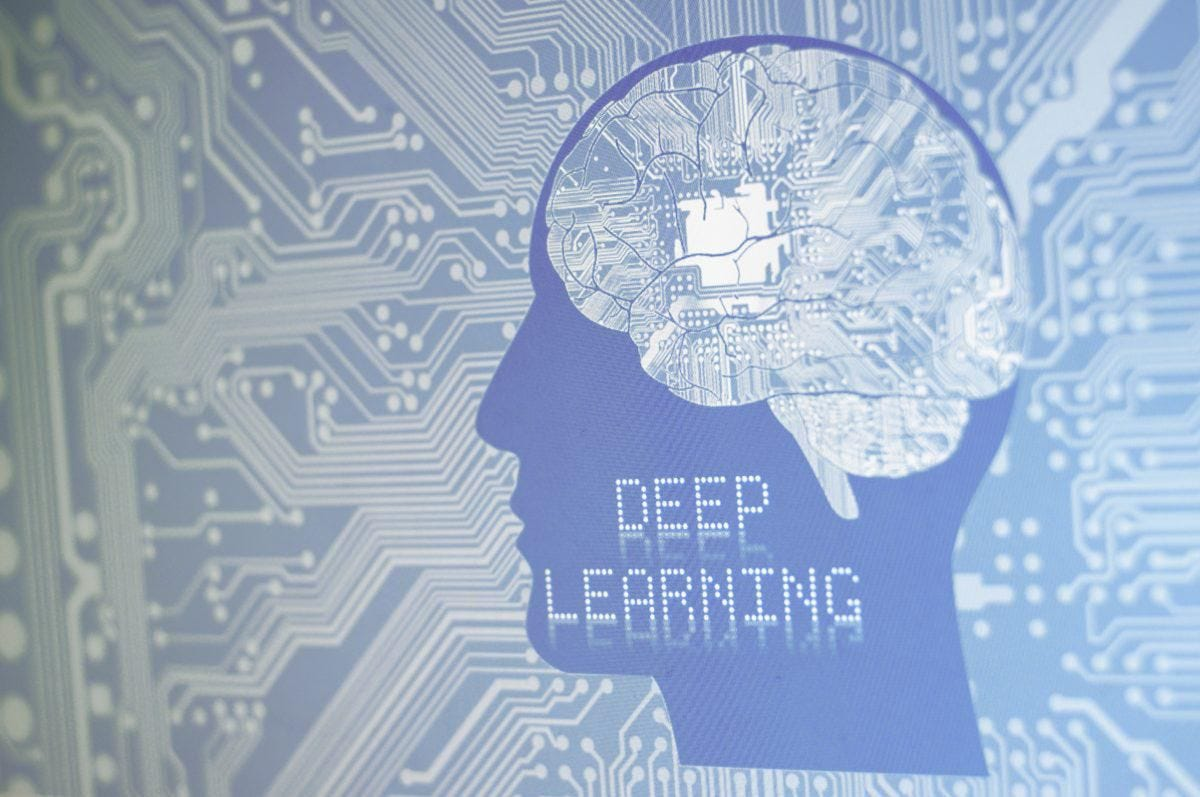# Quantization on PytorchLast story we talked about 8-bit quantization on PyTorch. PyTorch provides three approaches to quantize models. The first one is Dynamic quantization. The second is Post-Training static quantization. And the last is quantization aware training. Today let’s talk about weight and feature quantization during training.

A common approach to training quantized networks is to train in floating-point and then quantize the resulting weights. However, this may cause two common failure modes: 1) differences between full precision models with quantized models in ranges of weights for different output channels and 2) outlier values in quantized models. This paper proposed an approach that simulates quantization effects in the forward pass of training which can avoid the failures.

Weights and features(activations) can be treated in different formats to quantize. Weights are quantized before they are convolved with the input. Features are quantized at points where they would be during inference.

For each layer, quantization can be done by symmetric quantization and asymmetric quantization.

Symmetric Quantization

The formula for symmetric quantization is as follows:

Delta means the quantized scaling factor, x and xint respectively represent the value before and after quantization. The original floating-point data is quantized into a cell by dividing by the scaling factor and then rounding.

The corresponding dequantization formula is as follows:

The scaling factor is obtained according to the following formula:

rmin and rmax respectively represent the range of data before quantization.

Asymmetric Quantization

Compared with symmetric quantization, asymmetric quantization has one more zero offset. A float32 number is asymmetrically quantized to one int8 number. The formula for asymmetric quantization is as follows:

The de-quantization operation is:

The scaling factor and zero offset are calculated as follows:

Implement in PyTorch

During network training, the forward and backward parts are the most important parts we need to care about. The scaling factor for the weights is the same as above. However, we will apply the Exponential Moving Average(EMA) for activation quantization. The formula is as follows:

`moving_max = moving_max * momenta + max(abs(activation)) * (1- momenta)`

The momenta is closed to 1. I set it to 0.99 in the following experiments. And the scaling factor is moving_max/128.

Then the formula for the backpropagation is as follows:

The code for this part is as follows. Note that in this experiment we use float32 to simulate int8.

`class Quantizer(nn.Module):    def __init__(self, bits, range_tracker):        super().__init__()        self.bits = bits        self.range_tracker = range_tracker        self.register_buffer('scale', None)      # Scaling factor        self.register_buffer('zero_point', None) # zero offset    def update_params(self):        raise NotImplementedError    # Quantize    def quantize(self, input):        output = input * self.scale - self.zero_point        return output    def round(self, input):        output = Round.apply(input)        return output    # Clamp    def clamp(self, input):        output = torch.clamp(input, self.min_val, self.max_val)        return output    # De-quantize    def dequantize(self, input):        output = (input + self.zero_point) / self.scale`

Based on this, we implemented two schemes of symmetric and asymmetric quantization. For the quantization of the weight, it is better to obtain the scaling factor in different channels, and then obtain a scaling factor of the activation value among the whole network.

The implementation of this part is as follows:

`# ********************* range_trackers *********************class RangeTracker(nn.Module):    def __init__(self, q_level):        super().__init__()        self.q_level = q_level    def update_range(self, min_val, max_val):        raise NotImplementedError    @torch.no_grad()    def forward(self, input):        if self.q_level == 'L':    # A,min_max_shape=(1, 1, 1, 1),layer level            min_val = torch.min(input)            max_val = torch.max(input)        elif self.q_level == 'C':  # W,min_max_shape=(N, 1, 1, 1),channel level            min_val = torch.min(torch.min(torch.min(input, 3, keepdim=True), 2, keepdim=True), 1, keepdim=True)            max_val = torch.max(torch.max(torch.max(input, 3, keepdim=True), 2, keepdim=True), 1, keepdim=True)        self.update_range(min_val, max_val)class GlobalRangeTracker(RangeTracker):      def __init__(self, q_level, out_channels):        super().__init__(q_level)        self.register_buffer('min_val', torch.zeros(out_channels, 1, 1, 1))        self.register_buffer('max_val', torch.zeros(out_channels, 1, 1, 1))        self.register_buffer('first_w', torch.zeros(1))    def update_range(self, min_val, max_val):        temp_minval = self.min_val        temp_maxval = self.max_val        if self.first_w == 0:            self.first_w.add_(1)            self.min_val.add_(min_val)            self.max_val.add_(max_val)        else:            self.min_val.add_(-temp_minval).add_(torch.min(temp_minval, min_val))            self.max_val.add_(-temp_maxval).add_(torch.max(temp_maxval, max_val))class AveragedRangeTracker(RangeTracker):      def __init__(self, q_level, momentum=0.1):        super().__init__(q_level)        self.momentum = momentum        self.register_buffer('min_val', torch.zeros(1))        self.register_buffer('max_val', torch.zeros(1))        self.register_buffer('first_a', torch.zeros(1))    def update_range(self, min_val, max_val):        if self.first_a == 0:            self.first_a.add_(1)            self.min_val.add_(min_val)            self.max_val.add_(max_val)        else:            self.min_val.mul_(1 - self.momentum).add_(min_val * self.momentum)            self.max_val.mul_(1 - self.momentum).add_(max_val * self.momentum)`

The training part of the simulation quantization is implemented as follows:

`class Conv2d_Q(nn.Conv2d):    def __init__(        self,        in_channels,        out_channels,        kernel_size,        stride=1,        padding=0,        dilation=1,        groups=1,        bias=True,        a_bits=8,        w_bits=8,        q_type=1,        first_layer=0,    ):        super().__init__(            in_channels=in_channels,            out_channels=out_channels,            kernel_size=kernel_size,            stride=stride,            padding=padding,            dilation=dilation,            groups=groups,            bias=bias        )        if q_type == 0:            self.activation_quantizer = SymmetricQuantizer(bits=a_bits, range_tracker=AveragedRangeTracker(q_level='L'))            self.weight_quantizer = SymmetricQuantizer(bits=w_bits, range_tracker=GlobalRangeTracker(q_level='C', out_channels=out_channels))        else:            self.activation_quantizer = AsymmetricQuantizer(bits=a_bits, range_tracker=AveragedRangeTracker(q_level='L'))            self.weight_quantizer = AsymmetricQuantizer(bits=w_bits, range_tracker=GlobalRangeTracker(q_level='C', out_channels=out_channels))        self.first_layer = first_layer    def forward(self, input):        # Quantization for Features and Weights        if not self.first_layer:            input = self.activation_quantizer(input)        q_input = input        q_weight = self.weight_quantizer(self.weight)         # Quantization for Conv        output = F.conv2d(            input=q_input,            weight=q_weight,            bias=self.bias,            stride=self.stride,            padding=self.padding,            dilation=self.dilation,            groups=self.groups        )        return output`

I did some experiments on CIFAR10 dataset. The accuracy of the original model is 91%. The symmetric model achieved 89% and the asymmetric model can achieve 89%. There is still a 2% loss of accuracy. Currently, I don’t know the reason for this result.

Some accuracy of some classification networks provided on the paper is as follows:

Summary

Today we talked about how to do quantization in PyTorch and implemented the symmetric and asymmetric quantization. Compared with the results in the paper, there are still some performance gaps. In the future, we will talk more about how to improve the quantization process and other optimization for deep learning models. See you next time!